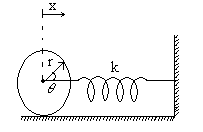Simple hormonic motion
Question

# A solid cylinder of mass M and radius R is connected to spring of force constant k as shown in fig. Cylinder rolls without slipping on the horizontal  surface. Its time period of oscillation isDifficult
Solution

## Total energy  $\frac{1}{2}{\mathrm{Mu}}^{2}\left(1+\frac{{\mathrm{K}}^{2}}{{\mathrm{R}}^{2}}\right)+\frac{1}{2}{\mathrm{Kx}}^{2}=\text{constant}$$\frac{1}{2}{\mathrm{Mu}}^{2}\left(1+\frac{1}{2}\right)+\frac{1}{2}{\mathrm{Kx}}^{2}=\text{constant}$$\mathrm{Mu}\frac{\mathrm{du}}{\mathrm{dt}}\left(\frac{3}{2}\right)+\mathrm{Kx}\frac{\mathrm{dx}}{\mathrm{dt}}=0$$\mathrm{Mu}\left(\frac{\mathrm{du}}{\mathrm{dt}}\right)\left(\frac{3}{2}\right)+\mathrm{Kxu}=0$$\frac{3\mathrm{M}}{2}\mathrm{a}+\mathrm{Kx}=0$

Get Instant Solutions